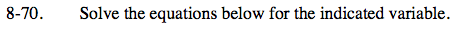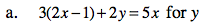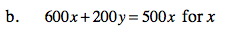Home > ACC7 > Chapter cc38 > Lesson cc38.2.2 > Problem8-70

8-70.
1. Solve the equations below for the indicated variable. Homework Help ✎

1. 3(2x − 1) + 2y = 5x for y

2. 600x + 200y = 500x for x3(2x) − 3(1) + 2y = 5x Distribute.
6x − 3 + 2y = 5x

-6x -6x Subtract 6x from both sides.
−3 + 2− = −x

+3 +3 Add 3 to both sides
2y = -x + 3

Divide both sides by 2.

${\it y} = \frac{-{\it x} + 3}{2}$Follow the same method as in part (a).